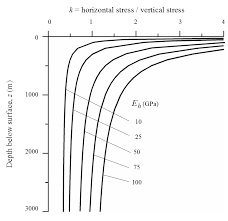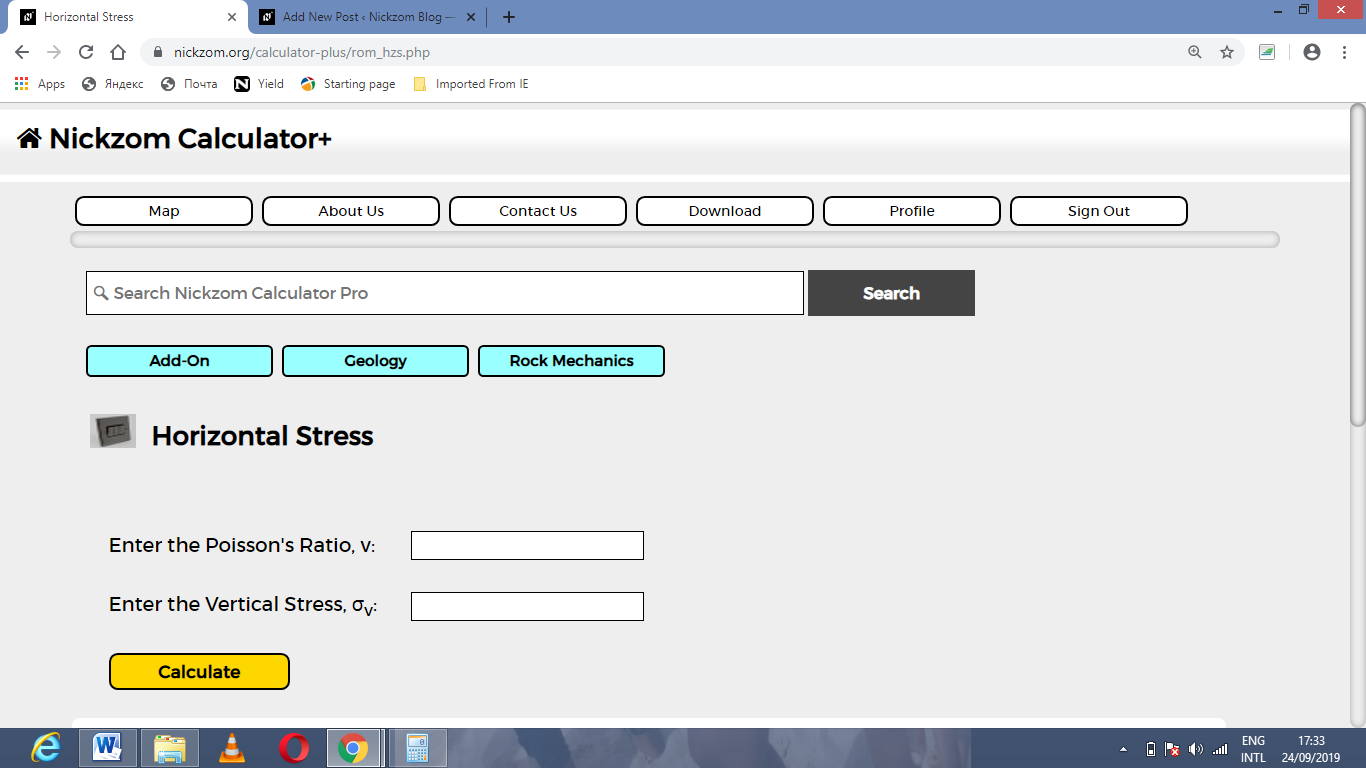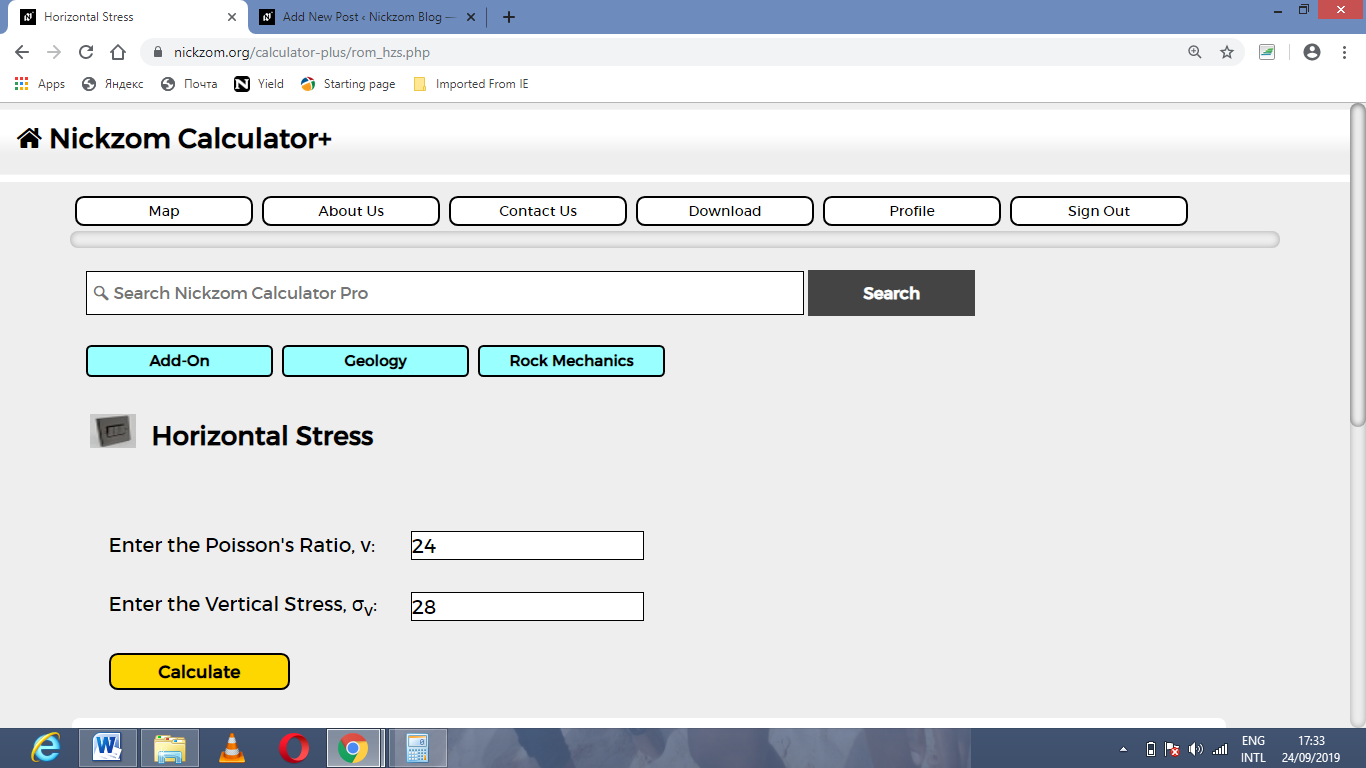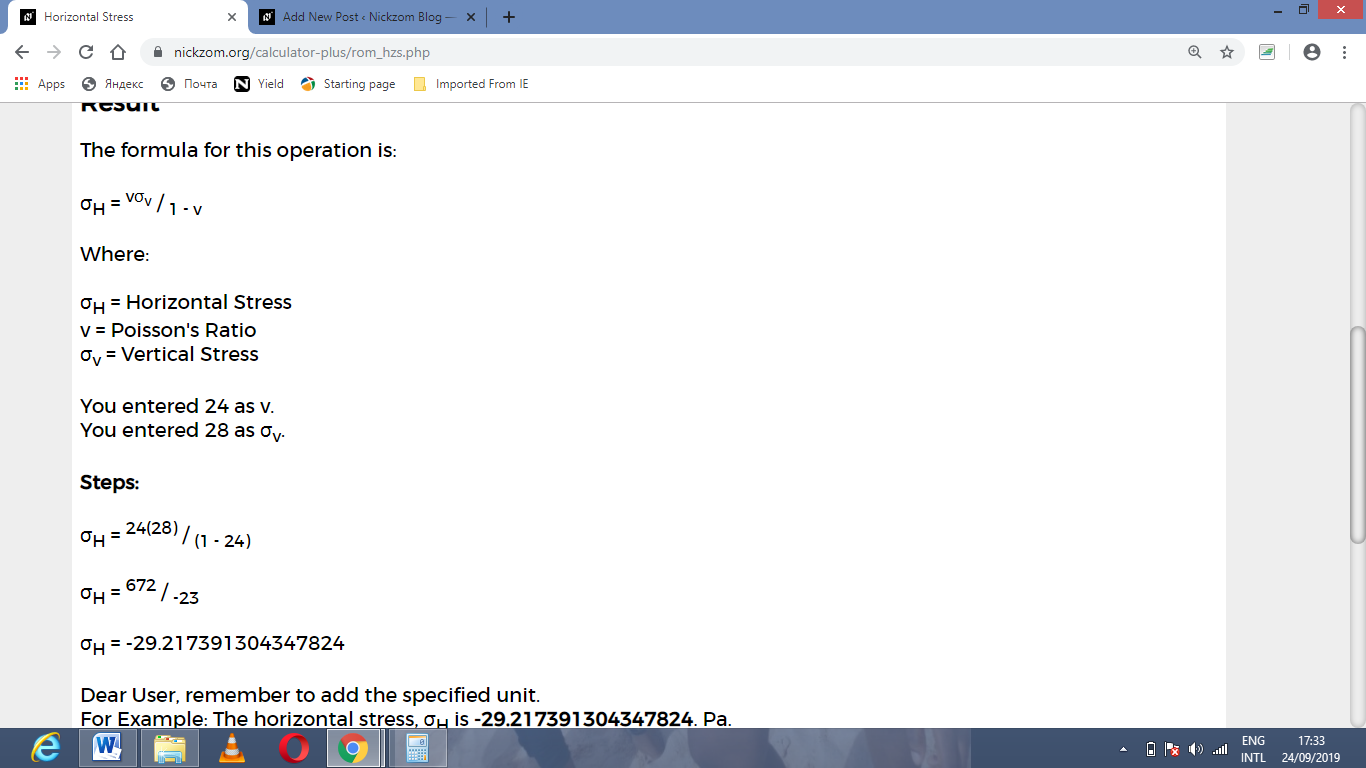# How to Calculate and Solve for Horizontal Stress | Rock MechanicsThe image above represents horizontal stress.

To compute for horizontal stress, two essential parameters are needed and these parameters are Poisson’s ratio (v) and vertical stress (σv).

The formula for calculating the horizontal stress:

σH = v / 1 – v

Where;

σH = Horizontal Stress
v = Poisson’s Ratio
σv = Vertical Stress

Let’s solve an example;
Find the horizontal stress when the Poisson’s ratio is 24 and the vertical stress is 28.

This implies that;

v = Poisson’s Ratio = 24
σv = Vertical Stress = 28

σH = v / 1 – v
σH = 24(28) / (1 – 24)
σH = 672 / -23
σH = -29.217

Therefore, the horizontal stress is 29.217.

Calculating the Poisson’s Ratio when the Horizontal Stress and Vertical stress is Given.

v = σH / σv + σH

Where;

v = Poisson’s Ratio
σH = Horizontal Stress
σv = Vertical Stress

Let’s solve an example;
Find the Poisson’s ratio when the horizontal stress is 12 and the vertical stress is 16.

This implies that;

σH = Horizontal Stress = 12
σv = Vertical Stress = 16

v = σH / σv + σH
v = 12 / 16 + 12
v = 12 / 28
v = 12 / 28
v = 0.428

Therefore, the Poisson’s ratio is 0.428.

Calculating the Vertical Stress when the Horizontal Stress and the Poisson’s Ratio is Given.

σv = σH (1 – v) / v

Where;

σv = vertical stress
σH = Horizontal Stress
v = Poisson’s ratio

Let’s solve an example;
Find the vertical stress when the horizontal stress is 14 and the Poisson’s ratio is 8.

This implies that;

σH = Horizontal Stress = 14
v = Poisson’s ratio = 8

σv = σH (1 – v) / v
σv = 14 (1 – 8) / 8
σv = 14 (- 7) / 8
σv = – 98 / 8
σv = – 12.25

Therefore, the vertical stress is – 12.25.

Nickzom Calculator – The Calculator Encyclopedia is capable of calculating the horizontal stress.

To get the answer and workings of the horizontal stress using the Nickzom Calculator – The Calculator Encyclopedia. First, you need to obtain the app.

You can get this app via any of these means:

To get access to the professional version via web, you need to register and subscribe for NGN 1,500 per annum to have utter access to all functionalities.
You can also try the demo version via https://www.nickzom.org/calculator

Apple (Paid) – https://itunes.apple.com/us/app/nickzom-calculator/id1331162702?mt=8
Once, you have obtained the calculator encyclopedia app, proceed to the Calculator Map, then click on Geology under Add-on.Now, Click on Rock Mechanics under GeologyNow, Click on Horizontal Stress under Rock MechanicsThe screenshot below displays the page or activity to enter your values, to get the answer for the lateral strain according to the respective parameters which are the Poisson ratio (v) and vertical stress (σv).Now, enter the values appropriately and accordingly for the parameters as required by the Poisson ratio (v) is 24 and vertical stress (σv) is 28.Finally, Click on CalculateAs you can see from the screenshot above, Nickzom Calculator– The Calculator Encyclopedia solves for the horizontal stress and presents the formula, workings and steps too.